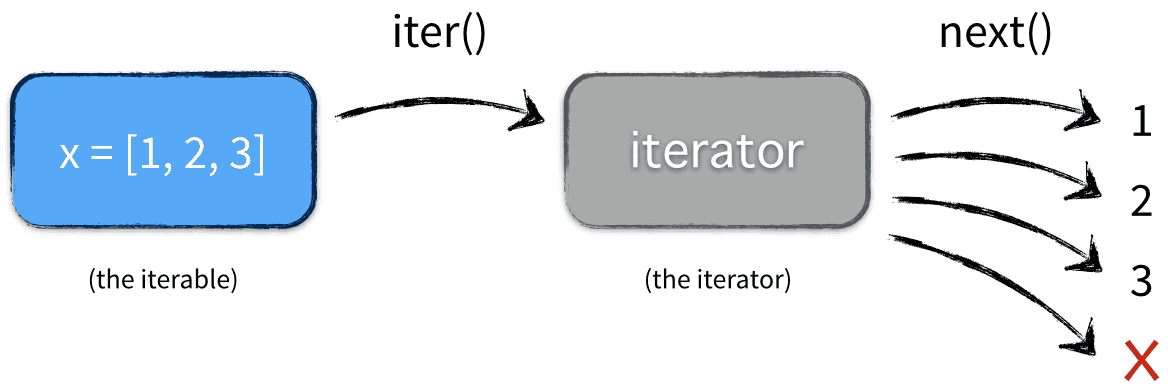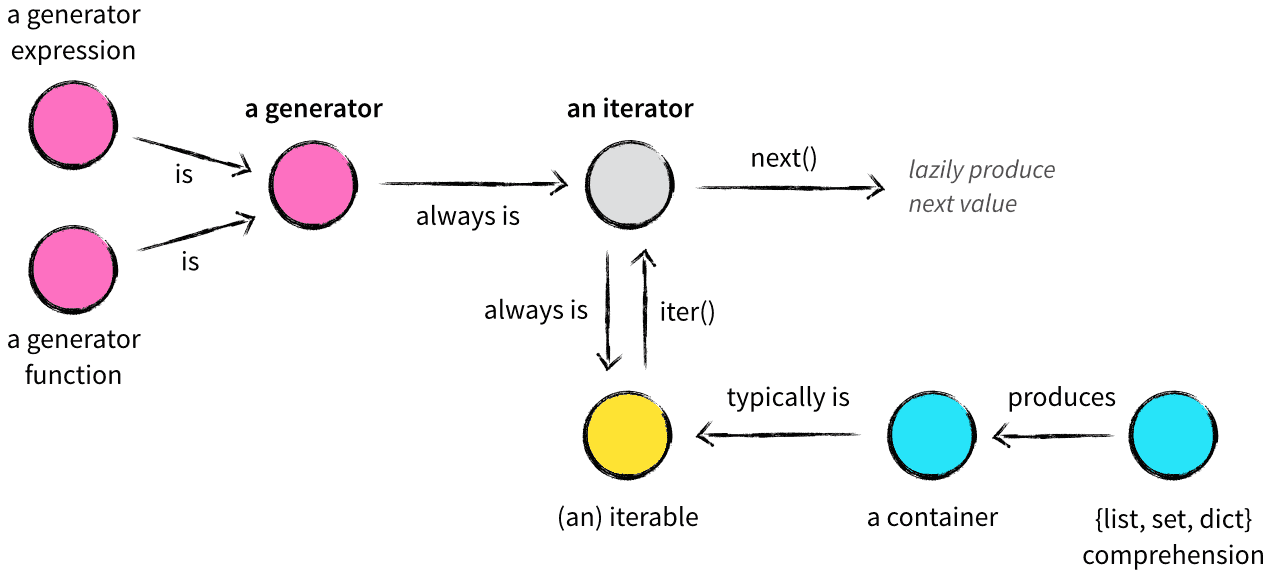# Python基础（四）——迭代器/对象，生成器 – 孔胡子

首先廖雪峰网站写的内容就我目前初步学习来说，已经相当详实，知识点平铺直叙让人易接受，所以以下内容均作为一种摘记记录以及补充

# 1. 列表生成器

主要目的是创建 list 。多看例子就能清楚：

```print(list(range(1,10,2)))  #[1, 3, 5, 7, 9].生成1~9（左闭右开）,相隔为2
print([t * t for t in range(1,10,3) if t % 2 == 0]) #.生成1~9相隔4,且是偶数的平方和
print([m + n for m in '123' for n in '456']) #['14', '15', '16', '24', '25', '26', '34', '35', '36'].全排列

d = {'x': 'A', 'y': 'B', 'z': 'C'}
for k, v in d.items():
print(k, '=', v)

print([m + '=' + n for m,n in d.items()])  #['x=A', 'y=B', 'z=C']

#筛选单词，并全变小写
L = ['Hello', 'World', 18, 'Apple', None]
print([t.lower() for t in L if isinstance(t, str)])  #['hello', 'world', 'apple']```

# 2. Iterable vs Iterator

iterable 是可迭代对象，iterator 是迭代器。两者都是 collection.abc 中得抽象类。iterator 继承自 iterable 。

• 　　iterable 有常见得 list，dict，str，tuple 等或者自定义的类（该类必须实现抽象方法 _iter()_）。当一个可迭代对象作为参数调用自身的 iter() 方法时，会返回一个迭代器。迭代器拥有 _next()_ 抽象方法，可迭代对象没有，通过该方法就可以逐个得到 “序列” 中的各个值，不断调用 _next()_ 方法，最后会引起 StopIteration 异常报错，代表迭代结束了。同时迭代器还拥有 _iter()_ 方法，所以迭代器也是个可迭代对象。即所有的迭代器都是可迭代对象，但是可迭代对象并不都是迭代器，基本判断方法是是否调用 next() 方法，list，dect，str，tuple 都并不行，即不是迭代器。

我们可以通过 isinstance 来判断：

```from collections.abc import Iterable,Iterator
t = [1,2,3]   #列表
print(isinstance(t, Iterable)) #true
print(isinstance(t, Iterator)) #false```

我们常用的 for…in [ ] 。就是利用了迭代器

```from collections.abc import Iterable,Iterator
L = [1,2,3]
print(isinstance(L, Iterator)) #Flase
for t in L:
print(t, end=' ') #1  2  3```

这是我们常写的代码，输出123。既然 L 不是迭代器为啥也能迭代输出呢。这就是在使用 for…in 的时候，Python 解释器主动将可迭代对象调用了 iter() 返回迭代器，即每次都是通过迭代器的 next() 方法进行输出。那么哪个异常 StopIteration 呢？异常应该被 for…in 内部处理了，并不显式的抛出。

我们换一种更明显的写法：

```1 from collections.abc import Iterable,Iterator
2 L = [1,2,3]
3 print(isinstance(L, Iterator))  #false
4 T = L.__iter__()
5 print(isinstance(T, Iterator))  #true。现在T就是迭代器了，拥有了next()方法。
6 print(T.__next__())  # 1
7 print(T.__next__())  # 2
8 print(T.__next__())  # 3
9 print(T.__next__())  # StopIteration```

结果和我们想的是一样的。或者再这样写

``` 1 from collections.abc import Iterable,Iterator
2 L = [1,2,3]
3 print(isinstance(L, Iterator))  #false
4 T = iter(L)
5 while True:
6     try:
7         print(next(T), end=' ')
8     except StopIteration:
9         print('结束')
10         break```

（点击图片查看原文）# 3. 生成器和 yield

• 生成器是返回一个 generator iterator 的函数。但是这个函数中包含 yield 表达式，除此之外别无它异，用来产生一系列供 for 循环使用的值或者通过 next() 逐一获取。所以生成器一般也称为生成器函数。
• 生成迭代器 generator iterator 是由生成器 generator 创建的对象。每遇到 yield 会暂停（相当于return），并记住当前位置，之后在继续在记住的位置继续向下运行。而不同于普通函数每次都由上往下运行。
• 第一种创建生成器的方法：将列表生成式的 [ ] 换成 ( )
```L = ( t * t for t in range(1,10))
print(L)  #<generator object <genexpr> at 0x0000028D2F68B840>
print(next(L)) # 1
print(next(L)) # 4
for i in L: # 迭代输出
print(i)```

• 当一个生成函数被调用时，返回一个迭代器，成为生成器。这个生成器来控制生成函数的执行，遇到 yield 就挂起，下次继续从 挂起处执行。前面说过迭代器有 next() 方法，所以这里的yield 就是干了 next() 方法的事。一样不断next 直到无数据 StopIteration。
• 第二种是通过定义函数
``` 1 def test():
2     print('1')
3     yield
4     print('2')
5     yield
6     print('3')
7     yield
8
9 t = test()  # t 是生成器，生成器来控制函数
10 print(t) # <generator object test at 0x0000021EF6C5B840>
11 next(t) # 1
12 next(t) # 2
13 next(t) # 3
14 next(t) # StopIteration```

可以通过 11~14 行看出，yield 起的作用就是挂起。第一次调用next() 方法，函数执行到第三句就停了，第二次调用 next() 执行到第五句。yield 就像是 OS 中的中断语句，保护现场–恢复现场。

再来看一个斐波那契例子：

``` 1 #斐波那契数列
2 #常规写法一：
3 # def fib(max):
4 #     n, a, b = 0, 0, 1
5 #     while n < max:
6 #         print(b)
7 #         a, b = b, a + b
8 #         n = n + 1
9 #     return 'done'
10 #
11 # fib(6)
12
13 #生成器写法二：
14 from collections.abc import Iterator,Iterable
15 def fib(max):
16     n, a, b = 0, 0, 1
17     while n < max:
18         yield b     # yield 类似于return 将 b 返回
19         a, b = b, a + b
20         n = n + 1
21     return 'done'
22
23 f = fib(6)
24 print(f)  #<generator object fib at 0x00000124DDD8B840>
25 print(isinstance(f, Iterator)) #True。生成函数返回迭代器
26
27 for n in f:
28     print(n, end=' ')  #1 1 2 3 5 8```

通过观察24，25行可以知道，调用了 fib() 之后，函数并没有执行到尾（否则返回 str = ‘done’），正如上文所说，返回的是一个 生成器，也就是调用生成函数（含 yield语句的）返回生成器，然后我们通过生成器来控制函数的执行。只有执行27行的 for…in 的时候，才会去执行15~21这段函数代码。

具体执行过程：第一次从16至18行停止，因为yield的存在，执行到18行就停了，然后返回一个值 b 给 for 循环，然后执行28行输出 b，然后next()迭代器继续从上次停止的地方的下一行19行继续执行(迭代器next()只要不是StopIteration 或者生成函数结束了，for 循环就得以继续)，然后在while循环内，再次执行到18行停止，返回 b 给 for。继续重复，直至跳出while循环，fib() 这段生成器函数结束了，for…in 也就结束了。

``` 1 # 生成器写法三
2 from collections.abc import Iterator,Iterable
3 def fib(max):
4     n, a, b = 0, 0, 1
5     while n < max:
6         yield b     # yield 类似于return 将 b 返回
7         a, b = b, a + b
8         n = n + 1
9
10 f = fib(3)
11 print(f)  #<generator object >
12 print(isinstance(f, Iterator)) #True。生成函数返回迭代器
13 print(next(f))  # 1
14 print(next(f))  # 1
15 print(next(f))  # 2
16 print(next(f))  # StopIteration```

# 4.生成器send()方法

stackoverflow 上还有关于生成 yield 配合使用 send()的方法。查阅官网，send(value) 函数意思：恢复执行，并向生成器发送一个值，value 参数将被当作 yield 表达式结果。

```1 def test():
2     while True:
3         x = yield
4         yield x * 2
5
6 g = test()
7 print(next(g)) # none
8 print(g.send(12)) #24```

我们已经知道 yield 可以当作return来理解。

首先第六行创建了 g (生成器)，第七行输出 none，因为执行第七行，也就是去执行test()函数了，函数顺利执行到第三行，3 = yield 明显是我们学的赋值语句，难道是将yield赋值给3？不是的。先解释输出的none，因为没有参数写在yield的右边，即没有参数返回，所以第七行输出 None。同时因为yield存在而停止继续。

而第八行：遇到g.send() 会继续执行上次执行到第三行的地方，这里传入的参数12就是赋值给x的。所以再往下第四行，yiled看成return 返回12*2，同时test()函数被挂起，返回24给第八行。至此函数结束。

又比如：

```1 def test(x):
2     while True:
3         x *= 2
4         x = yield x
5
6 g = test(3)
7 print(next(g)) # 6
8 print(g.send(12))  # 24```

第四行意思：先看右边yield x 就是返回 x 。再看左边 x = yield 就是赋值给 x 。所以不难理解了。不解释了。

# 5. 补充（点击图片查看原文）Copyright © All Rights Reserved · Green Hope Theme by Sivan & schiy · Proudly powered by WordPress